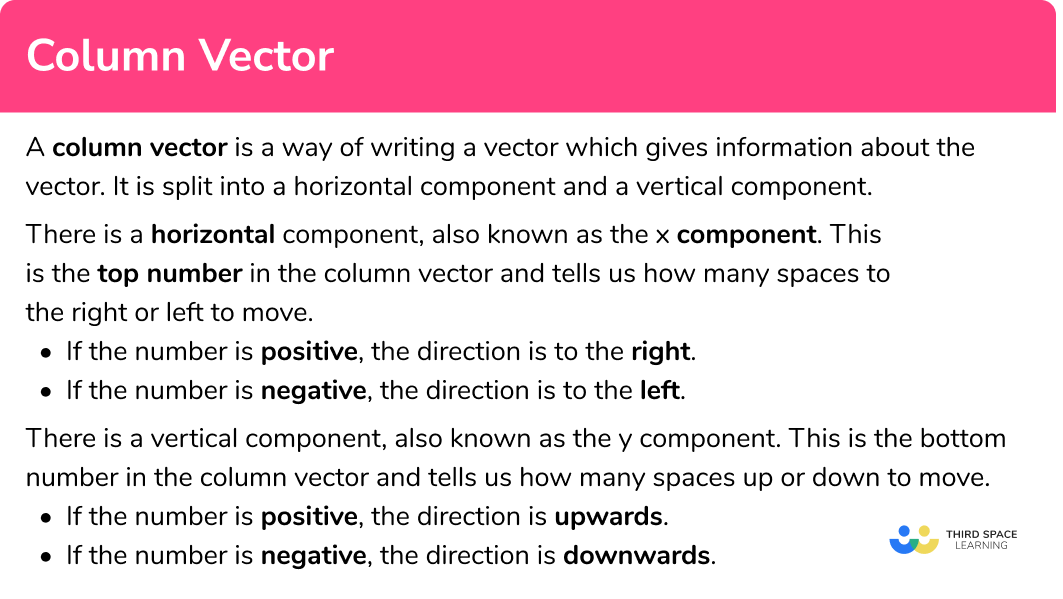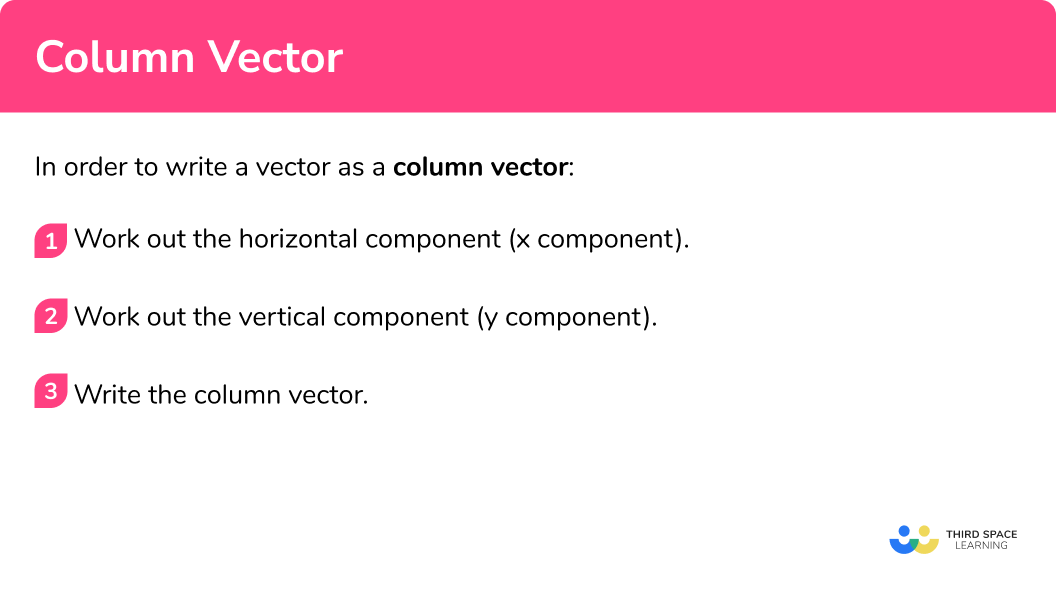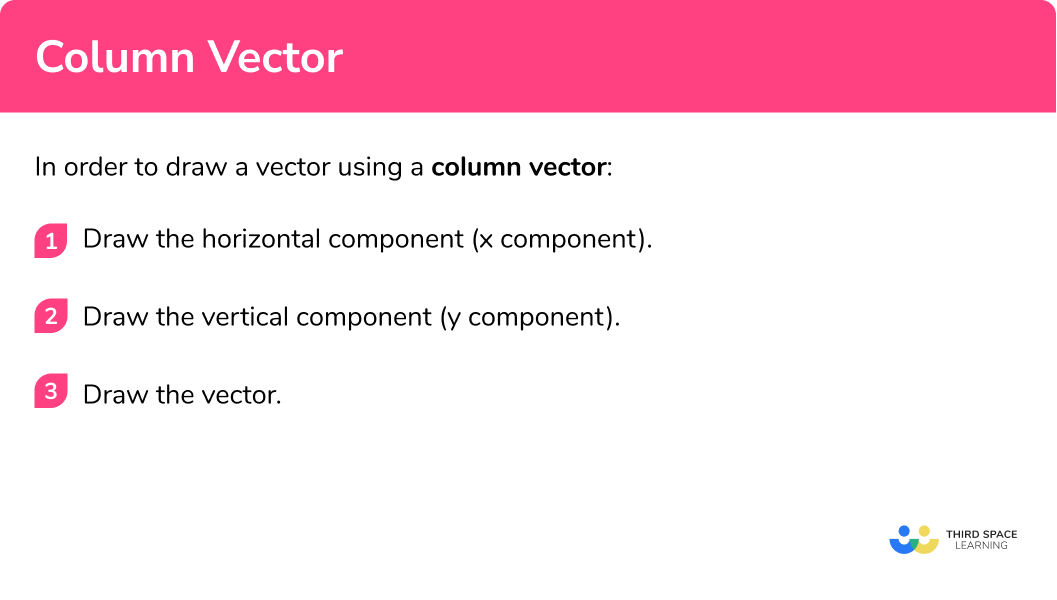# Column Vector

Here we will learn about column vectors, including how to write a column vector and how to draw a diagram of a column vector.

There are also vector worksheets based on Edexcel, AQA and OCR exam questions, along with further guidance on where to go next if you’re still stuck.

## What is a column vector?

A column vector is a way of writing a vector which gives information about the vector. It is split into a horizontal component and a vertical component.

There is a horizontal component, also known as the \textbf{x} component This is the top number in the column vector and tells us how many spaces to the right or left to move.

If the number is positive, the direction is to the right.

If the number is negative, the direction is to the left.

There is a vertical component, also known as the \textbf{y} component. This is the bottom number in the column vector and tells us how many spaces up or down to move.

If the number is positive, the direction is upwards.

If the number is negative, the direction is downwards.

E.g.

Vector \textbf{a} can be written as the column vector \begin{pmatrix} \; 3 \;\\ \; 2 \; \end{pmatrix}

\textbf{a}= \begin{pmatrix} \; 3 \;\\ \; 2 \; \end{pmatrix} \text{is} \begin{pmatrix} 3 \ \text{right}\\ 2 \ \text{up}\\ \end{pmatrix}

Notice the horizontal component and the vertical component make a right-angled triangle.

E.g.

Vector \textbf{b} can be written as the column vector \begin{pmatrix} \; 3 \;\\ \; -4 \; \end{pmatrix}

\textbf{b}= \begin{pmatrix} \; 3 \;\\ \; -4 \; \end{pmatrix} \text{is} \begin{pmatrix} 3 \ \text{right}\\ -4 \ \text{down}\\ \end{pmatrix}

Notice the horizontal component and the vertical component make a right-angled triangle.

### What is a column vector?## How to write a column vector

In order to write a vector as a column vector:

1. Work out the horizontal component ( \textbf{x} component).
2. Work out the vertical component ( \textbf{y} component).
3. Write the column vector.

### How to write a column vector## Related lessons on vectors

Column vector is part of our series of lessons to support revision on vectors. You may find it helpful to start with the main vectors lesson for a summary of what to expect, or use the step by step guides below for further detail on individual topics. Other lessons in this series include:

## Column vector examples

### Example 1: write a column vector

Write vector \textbf{a} as a column vector.

1. Work out the horizontal component ( \textbf{x} component).

From the starting point of the vector, draw a horizontal line.

This line is 4 squares to the right.

2Work out the vertical component ( \textbf{y} component).

From the end of the horizontal component, draw a vertical line to the end of the vector.

This line is 3 squares up.

3Write the column vector.

Write the horizontal component and the vertical component in a column vector.

Vector \textbf{a} as a column vector is:

\textbf{a}= \begin{pmatrix} \; 4 \;\\ \; 3 \; \end{pmatrix}

### Example 2: write a column vector

Write vector \textbf{b} as a column vector.

From the starting point of the vector, draw a horizontal line.

This line is 4 squares to the right.

From the end of the horizontal component, draw a vertical line to the end of the vector.

This line is 1 square down.

Write the horizontal component and the vertical component in a column vector.

Vector \textbf{b} as a column vector is:

\textbf{b}= \begin{pmatrix} \; 4 \;\\ \; -1 \; \end{pmatrix}

### Example 3: write a column vector

Write vector \textbf{v} as a column vector.

From the starting point of the vector, draw a horizontal line.  We are trying to make a right-angled triangle.

This line is 2 squares to the left.

From the end of the horizontal component, draw a vertical line to the end of the vector.

This line is 1 square down.

Write the horizontal component and the vertical component in a column vector.

Vector \textbf{v} as a column vector is:

\textbf{v}= \begin{pmatrix} \; -2 \;\\ \; -1 \; \end{pmatrix}

## How to draw a vector using a column vector

In order to draw a diagram of a column vector:

1. Draw the horizontal component ( \textbf{x} component).
2. Draw the vertical component ( \textbf{y} component).
3. Draw the vector.

### How to draw a vector using a column vector## Drawing a diagram of a column vector examples

### Example 4: draw a diagram of the column vector

Draw a diagram of the column vector \begin{pmatrix} \; 2 \;\\ \; 5 \; \end{pmatrix}

On the grid choose a starting point and draw the horizontal component

The top number is 2 so we draw a line 2 squares to the right .

From the end of the horizontal component, draw the vertical component.

The bottom number is 5 so we draw a line 5 squares up.

Join up the starting point and the end point and remember to put the direction arrow on the line.

### Example 5: draw a diagram of the column vector

Draw a diagram of the column vector \begin{pmatrix} \; -3 \;\\ \; 1 \; \end{pmatrix}

On the grid choose a starting point and draw the horizontal component

The top number is -3 so we draw a line 3 squares to the left .

From the end of the horizontal component, draw the vertical component.

The bottom number is 1 so we draw a line 1 square up.

Join up the starting point and the end point and remember to put the direction arrow on the line.

### Example 6: draw a diagram of the column vector

Draw a diagram of the column vector \begin{pmatrix} \; -6 \;\\ \; -1 \; \end{pmatrix}

On the grid choose a starting point and draw the horizontal component

The top number is -6 so we draw a line 6 squares to the left.

From the end of the horizontal component, draw the vertical component.

The bottom number is -1 so we draw a line 1 square down.

Join up the starting point and the end point and remember to put the direction arrow on the line.

### Common misconceptions

• Make sure the signs are correct

Remember:

If the top number is positive, the direction is to the right.

If the top number is negative, the direction is to the left.

If the bottom number is positive, the direction is upwards.

If the bottom number is negative, the direction is downwards.

• Column vectors notation

Column vectors only have 2 numbers within the brackets; a top number and a bottom number. There is no need for any other punctuation marks such as commas or semicolons and there is no need for a line to separate the numbers.

### Practice column vector questions

1. Write this vector as a column vector:\begin{pmatrix} \; -4 \;\\ \; 1 \; \end{pmatrix}\begin{pmatrix} \; 4 \;\\ \; 1 \; \end{pmatrix}\begin{pmatrix} \; 1 \;\\ \; 4 \; \end{pmatrix}\begin{pmatrix} \; -1 \;\\ \; 4 \; \end{pmatrix}Draw a horizontal line and a vertical line and count the squares.\begin{pmatrix} \; 4 \;\\ \; 1 \; \end{pmatrix}

2. Write this vector as a column vector:\begin{pmatrix} \; 2 \;\\ \; -4 \; \end{pmatrix}\begin{pmatrix} \; 4 \;\\ \; -2 \; \end{pmatrix}\begin{pmatrix} \; -4 \;\\ \; -2 \; \end{pmatrix}\begin{pmatrix} \; -2 \;\\ \; 4 \; \end{pmatrix}Draw a horizontal line and a vertical line and count the squares.\begin{pmatrix} \; -2 \;\\ \; 4 \; \end{pmatrix}

3. Write the vector \textbf{x} as a column vector:\begin{pmatrix} \; 1 \;\\ \; 3 \; \end{pmatrix}\begin{pmatrix} \; -1 \;\\ \; 3 \; \end{pmatrix}\begin{pmatrix} \; 1 \;\\ \; -3 \; \end{pmatrix}\begin{pmatrix} \; -1 \;\\ \; -3 \; \end{pmatrix}Draw a horizontal line and a vertical line and count the squares.The column vector for \textbf{x} is

\begin{pmatrix} \; 1 \;\\ \; -3 \; \end{pmatrix}

4. Draw the vector

\begin{pmatrix} \; 1 \;\\ \; 4 \; \end{pmatrix}The top number of the column vector is 1 . This is the horizontal component. Use this to draw a horizontal line to the right. The bottom number of the column vector is 4 . This is the vertical component. Use this to draw a vertical line upwards.5. Draw the vector

\begin{pmatrix} \; -3 \;\\ \; -2 \; \end{pmatrix}The top number of the column vector is -3 . This is the horizontal component. Use this to draw a horizontal line to the left. The bottom number of the column vector is -2 . This is the vertical component. Use this to draw a vertical line downwards.6. Draw the vector

\begin{pmatrix} \; -5 \;\\ \; 2 \; \end{pmatrix}The top number of the column vector is -5 . This is the horizontal component. Use this to draw a horizontal line to the left. The bottom number of the column vector is 2 . This is the vertical component. Use this to draw a vertical line upwards.### Column vector GCSE questions

1.  Which is the correct column vector for this vector?(1 mark)

\begin{aligned} &\quad \text{B} \\\\ &\begin{pmatrix} \; -4 \;\\ \; 0 \; \end{pmatrix} \end{aligned}

(1)

2.  The column vector \begin{pmatrix} \; 4 \;\\ \; a \; \end{pmatrix} represents:What is the value of a ?

(1 mark)

a=-3

(1)

3. Write the column vector for this vector(2 marks)

\begin{pmatrix} \; 2 \;\\ \; -5 \; \end{pmatrix}

(For the correct horizontal component)

(1)

(For the correct vertical component)

(1)

## Learning checklist

You have now learned how to:

• How to write a vector as a column vector
•  How to draw a diagram of a column vector

## Did you know?

Not covered in GCSE: we can transpose a column vector to write it as a row vector (and vice versa). These look like co-ordinates, but do not have commas.

Vectors can also be extended into A Level Maths and Further Maths by learning how to multiply two vectors together using the dot product.

Column vectors are a simple example of matrices.  In GCSE maths we have a single column. Matrices are studied in A Level Further Maths. The number of columns and rows will be more than 1 . Matrix multiplication can be studied along with finding the inverse of a matrix. We can also find the determinant of a matrix and go further and look into eigenvalues and eigenvectors.

## Still stuck?

Prepare your KS4 students for maths GCSEs success with Third Space Learning. Weekly online one to one GCSE maths revision lessons delivered by expert maths tutors.

Find out more about our GCSE maths tuition programme.